In this post, the global oil production and consumption data between 1980 and 2017, as reported in the BP statistical review of world energy 2018 report, are used to create different plots using ggplot2 package. In addition, the gganimate package is used to create an animated map for the US and Canada annual oil consumption data over time.

The project is divided into three parts:

2. Importing and cleaning data using dplyr, tidyr, and stringr packages (from tidyverse collection).
3. Creating different types of plots with ggplot2 (from tidyverse collection) and gganimate packages.

``````pkg_list <- c("tidyverse", "gganimate", "readxl", "maps", "mapproj", "transformr")

# only install a package from the pkg_list if it is not already installed.
for (package in pkg_list) {

if (!(package %in% installed.packages()[, "Package"])) {

install.packages(package)

}
}

for (package in pkg_list) {

if (!(require(package,character.only = TRUE))) {

require(package,character.only = TRUE)

}
}``````

### 2. Importing and cleaning data

The original data file is downloded in Excel format from BP website and is stored as bp_stats_review_2018_all_data.xlsx in the working directory of the project.

In the first step, the oil proved reserves data for different countries between 1980 and 2017 are read from the spreadsheet file.

``````oil_proved_reserves <- as.data.frame(read_excel("bp_stats_review_2018_all_data.xlsx", sheet = "Oil - Proved reserves history", range = "A3:AM73"))

names(oil_proved_reserves) <- "country"

oil_proved_reserves <- select(oil_proved_reserves,1:(ncol(oil_proved_reserves)))

oil_proved_reserves <- oil_proved_reserves[complete.cases(oil_proved_reserves),]``````

In the above chunk of code, all the data in the “Oil - Proved reserves history” tab from row 3 to row 73 is read first and then the format of data is changed to data frame. In the second line of the code, name of the first column is changed to “country”. All the columns in the data frame except the last three ones are selected in the third line of the code. Finally, all the rows with missing values (NAs) are removed from the data frame using complete.cases() function.

Next, a new column called “Region_Continent” is created with the information coming from the reported regions/continents in the first column of the data frame.

``````Region_index <- which(str_detect(oil_proved_reserves[,1] ,pattern = "Total"))

Region_name <- as.character(str_split(oil_proved_reserves[Region_index,1],pattern = " ", n = 2, simplify = TRUE)[,2])

Region_info <- bind_cols(as.data.frame(Region_index),as.data.frame(Region_name,stringsAsFactors = FALSE))

counter <- 1

temp <- Region_index

for (i in (1:tail(Region_index,1))) {

if (i < temp) {

oil_proved_reserves\$Region_Continent[i] <- as.character(Region_info[Region_index == temp,])

} else if (i == temp) {

counter <- counter  + 1

temp <- Region_index[counter]

oil_proved_reserves\$Region_Continent[i] <- ""

} else {

}
}``````

Before converting the format of data frame from wide to long, an additional cleaning step is performed.

``````# wide format

oil_proved_reserves <- oil_proved_reserves[-Region_index,]

oil_proved_reserves <- select(oil_proved_reserves,country,Region_Continent,as.character(1980:2017))

oil_proved_reserves[oil_proved_reserves == "n/a"] <- NA

oil_proved_reserves[3:ncol(oil_proved_reserves)] <- as.numeric(unlist(oil_proved_reserves[3:ncol(oil_proved_reserves)]))``````

Finally, the wide format is converted to a long format in order to generate useful timeseries plots.

``````# long format

oil_proved_reserves_long <- oil_proved_reserves %>% pivot_longer(names_to = "year", values_to = "oil_reserve", -c("country","Region_Continent"))

oil_proved_reserves_long\$year <- as.integer(oil_proved_reserves_long\$year)``````

### 3. Creating different types of plots with ggplot2

#### 3.1. Oil reserves plots

Plotting oil reserves data on a world map requires world map information including country names, latitude, and longitude.

``world_map <- ggplot2::map_data("world")``
``tail(world_map)``

Before binding the “oil_proved_reserves_long” and “world_map” data frames with matching country names, pre-screening country names in both data frames helps us detect any differences between reported names in the two data frames.

``unique(oil_proved_reserves_long\$country[!(oil_proved_reserves_long\$country %in% world_map\$region)])``
``````##   "US"                       "Trinidad & Tobago"
##   "Other S. & Cent. America" "United Kingdom"
##   "Other Europe"             "Russian Federation"
##   "USSR"                     "Other CIS"
##   "Other Middle East"        "Other Africa"
##  "Other Asia Pacific"``````

The above line of code shows that US, Trinidad & Tobago, Russian Federation, and United Kingdom are labeled differently in both data frames. Therefore, the reported names in the “oil_proved_reserves_long” data frame are changed accordingly in order to have identical names in both data frames.

``````oil_proved_reserves_long[oil_proved_reserves_long\$country == "US",]\$country <- "USA"

oil_proved_reserves_long[oil_proved_reserves_long\$country == "United Kingdom",]\$country <- "UK"

oil_proved_reserves_long[oil_proved_reserves_long\$country == "Russian Federation",]\$country <- "Russia"

A single data frame is created below by joining the two data frames.

``````world_reserves <- left_join(world_map, oil_proved_reserves_long, by = c('region' = 'country'))

world_reserves_2017 <- world_reserves %>% filter(year %in% c(NA,2017))

colnames(world_reserves_2017) <- "Reserves"``````

The “NA” argument inside the the filter() function keeps all the countries in the “world_reserves_2017” dataset (in other words, all countries with no reported reserves data are also shown on the world map with gray color).

``````ggplot() +
geom_map(data = world_reserves_2017, map = world_reserves_2017, aes(x = long, y = lat, map_id = region, fill = Reserves)) +
scale_fill_distiller(palette = "Spectral", na.value = "gray94") +
ggtitle("Source: BP Statistical Review of World Energy 2018. ") +
theme_bw() +
theme(axis.text = element_text(size = 10, face = "bold"),
axis.title = element_text(size = 10, face = "bold"),
axis.ticks = element_blank(),
panel.grid  = element_blank(),
legend.title = element_text(size = 12, face = "bold"),
plot.title = element_text(size = 8, face = "bold")) ``````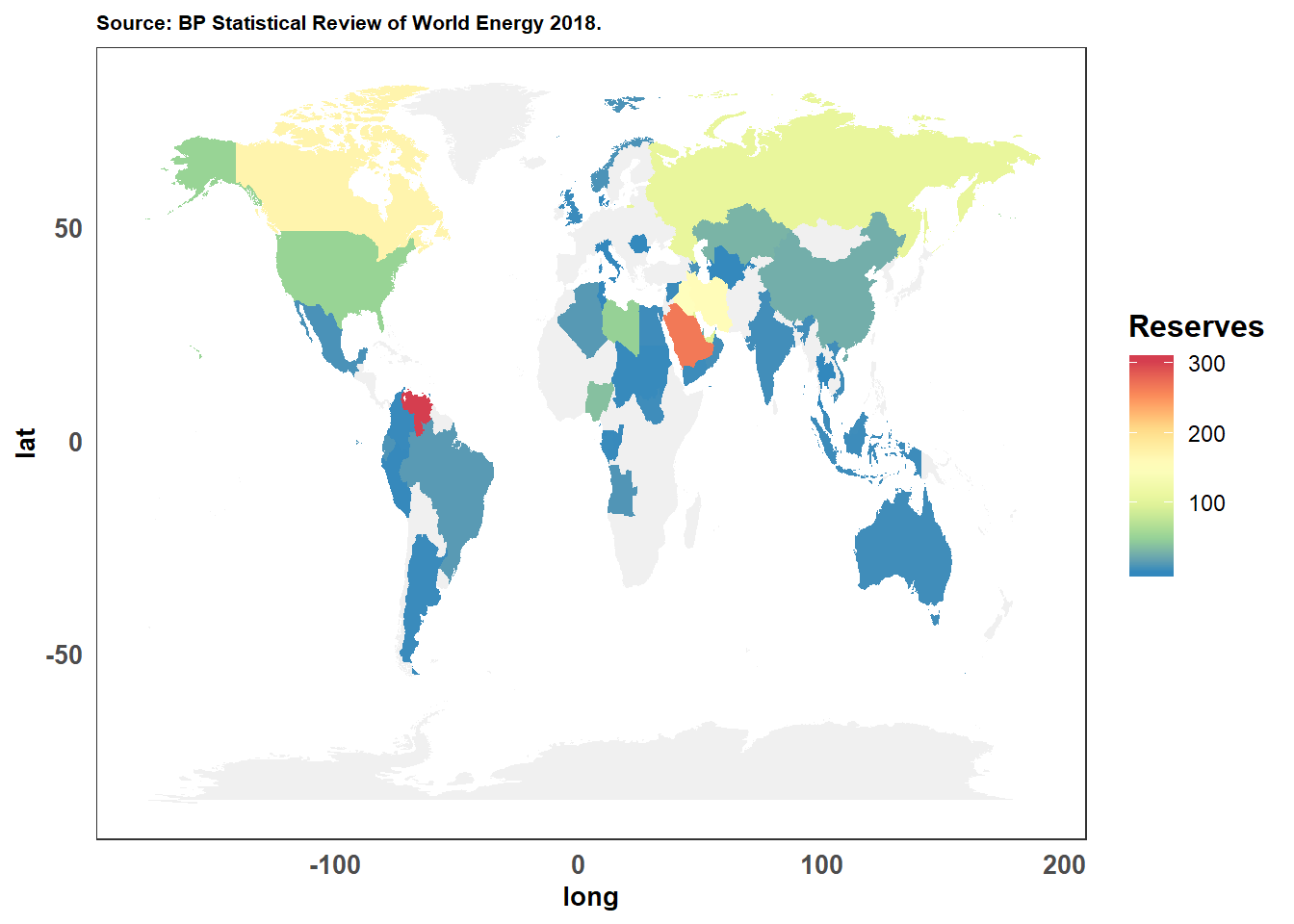World map of oil reserves at the end of 2017. There is either no data or lumped data for gray-colored countries.

The top 20 countries with the largest oil reserves in the world at the end of 2017 are shown below on a bar plot. The top 20 countries are selected by sorting the reserves data in descending order.

``````largest_reserves_2017 <- oil_proved_reserves_long %>% filter(year == 2017) %>% select(country,oil_reserve) %>% arrange(desc(oil_reserve))

country_ordered <- factor(largest_reserves_2017\$country, ordered = TRUE, levels = largest_reserves_2017\$country)

largest_reserves_2017\$country <- country_ordered

ggplot(largest_reserves_2017[c(1:20),],aes(x = country, y = oil_reserve, fill = country)) +
geom_col() +
scale_x_discrete(name = "") +
scale_y_continuous(name = "Oil Reserves (Thousand million barrels)", breaks = c(0, 50, 100, 150, 200, 250, 300)) +
ggtitle("Source: BP Statistical Review of World Energy 2018.") +
theme_bw() +
theme(axis.text = element_text(size = 10, face = "bold"),
axis.title = element_text(size = 10, face = "bold"),
axis.text.x = element_text(angle = 90, hjust = 1),
axis.ticks.x = element_blank(),
panel.grid  = element_blank(),
plot.title = element_text(size = 8, face = "bold"),
legend.position = "none") ``````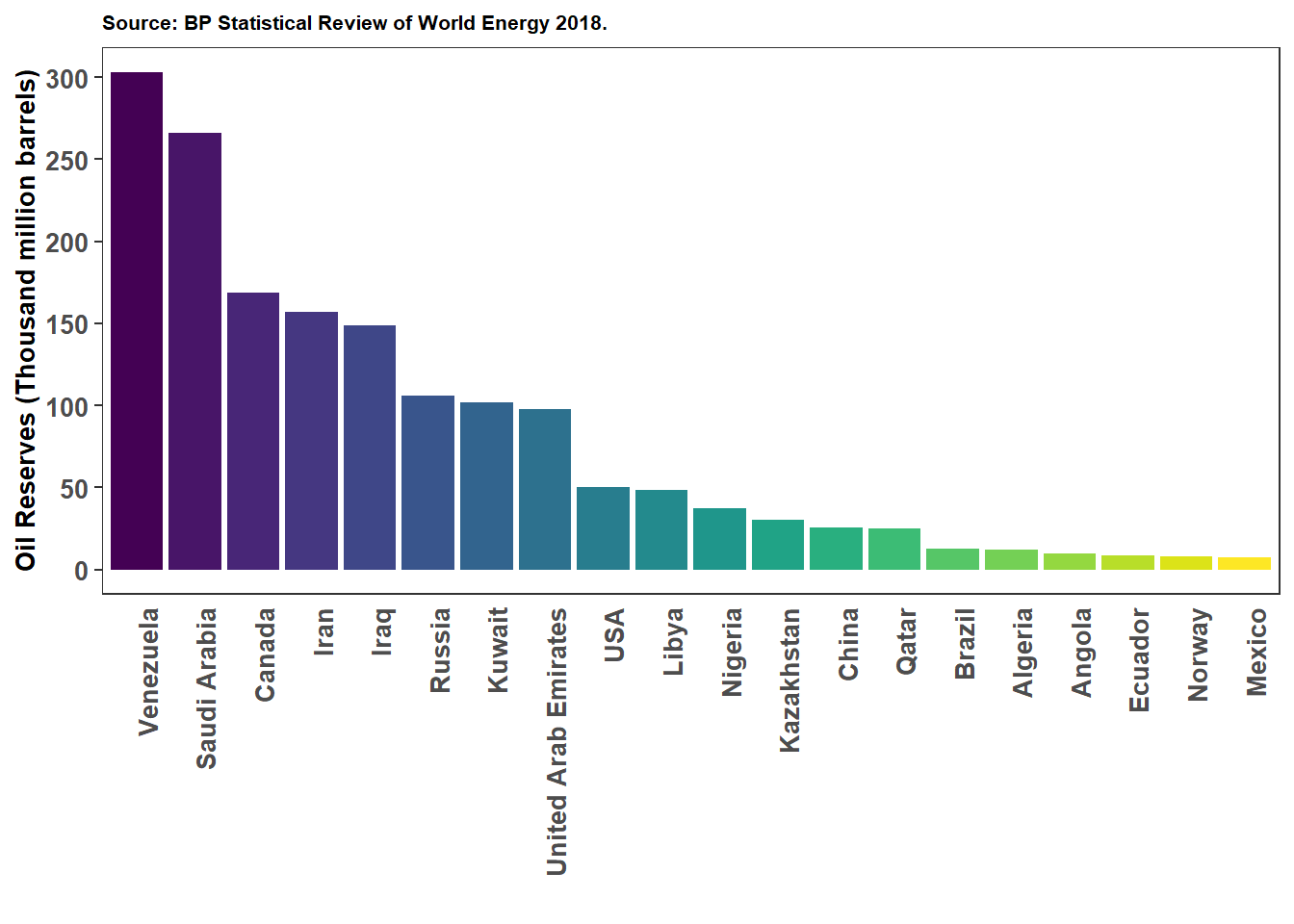The top 20 countries with the largest oil proved reserves at the end of 2017.

The following plot shows the oil reserves estimates in all the continents/regions of the world since 1980.

``````oil_reserves_1980_2017 <- oil_proved_reserves_long %>% group_by(Region_Continent, year) %>% summarise(continent_reserves = sum(oil_reserve, na.rm = TRUE))

colbrew2 <- c("#e41a1c", "#377eb8", "#4daf4a", "#984ea3", "#ff7f00", "#ffff33", "#a65628")

ggplot(oil_reserves_1980_2017, aes(x = year, y = continent_reserves, color = Region_Continent)) +
geom_line(size = 2) +
scale_color_manual(values = colbrew2) +
scale_x_continuous(name = "year", expand = c(0, 0), limits = c(1980, 2018), breaks = c(1980,1985,1990,1995,2000,2005,2010,2015,2018)) +
scale_y_continuous(name = "Oil reserves (Thousand million barrels)", limits = c(0, 900), expand = c(0, 0), breaks = c(0,100,200,300,400,500,600,700,800,900)) +
ggtitle("Source: BP Statistical Review of World Energy 2018.
*CIS: Commonwealth of Independent States.") +
theme_bw() +
theme(axis.text = element_text(size = 10, face = "bold"),
axis.title = element_text(size = 10, face = "bold"),
panel.grid.major =  element_line(colour = "grey50"),
axis.text.x = element_text(angle = 90, hjust = 1),
legend.title = element_text(size = 12, face = "bold"),
plot.title = element_text(size = 8, face = "bold")) ``````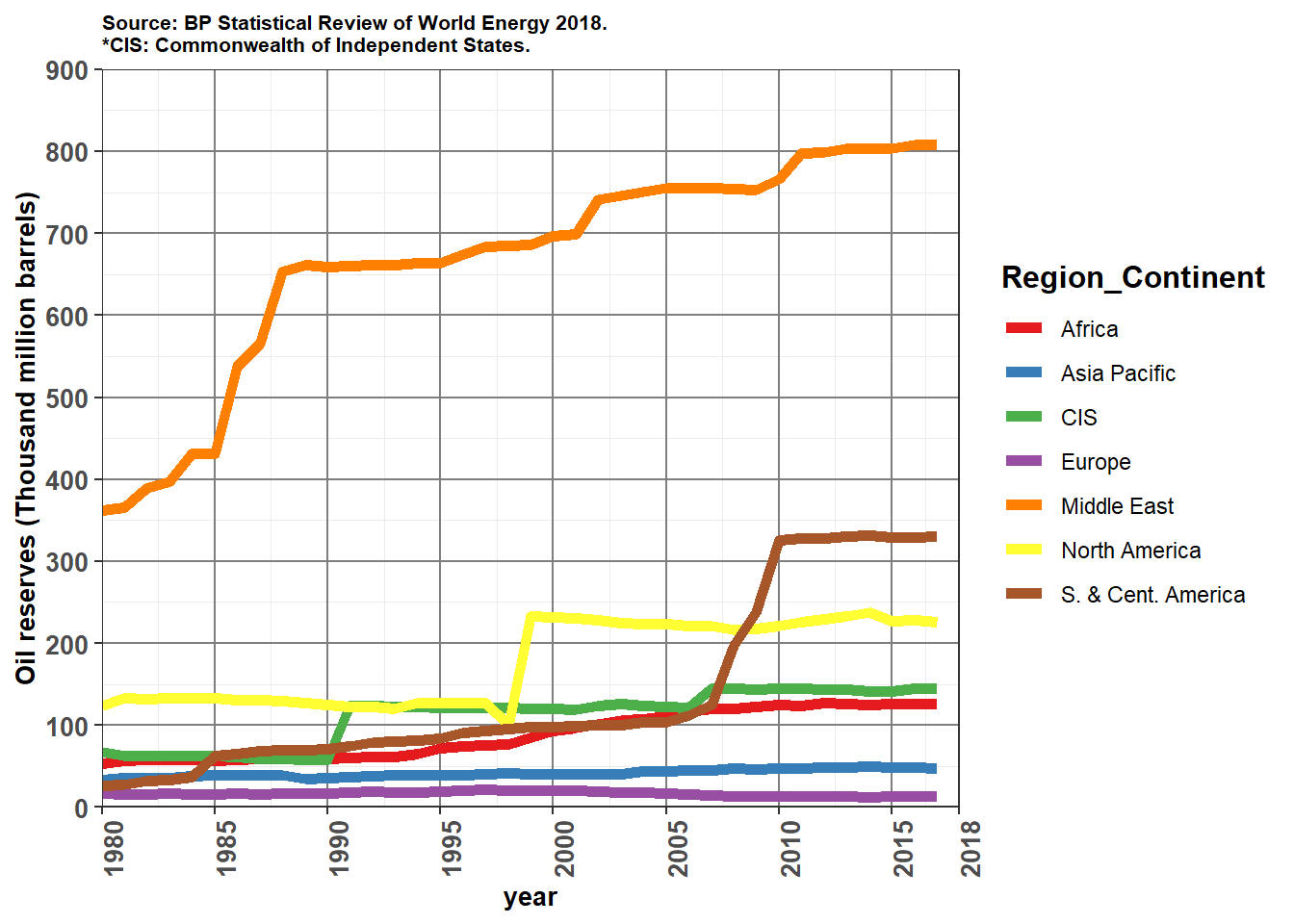Oil proved reserves. Color codes from colorbrewer2.org

#### 3.2. Oil production plots

The oil production data file in a long format is generated below.

``````oil_production <- as.data.frame(read_excel("bp_stats_review_2018_all_data.xlsx", sheet = "Oil Production - Barrels", range =  "A3:BB73"))

names(oil_production) <- "country"

oil_production <- select(oil_production,1:(ncol(oil_production)))

oil_production <- oil_production[complete.cases(oil_production),]

Region_index <- which(str_detect(oil_production[,1] ,pattern = "Total"))

Region_name <- as.character(str_split(oil_production[Region_index,1],pattern = " ", n = 2, simplify = TRUE)[,2])

Region_info <- bind_cols(as.data.frame(Region_index),as.data.frame(Region_name,stringsAsFactors = FALSE))

counter <- 1

temp <- Region_index

for (i in (1:tail(Region_index,1))) {
if (i < temp) {
oil_production\$Region_Continent[i] <- as.character(Region_info[Region_index == temp,])
} else if (i == temp) {
counter <- counter  + 1
temp <- Region_index[counter]
oil_production\$Region_Continent[i] <- ""
} else {
}
}

# Wide format
oil_production <- oil_production[-Region_index,]

oil_production <- select(oil_production,country,Region_Continent,as.character(1980:2017))

oil_production[oil_production == "n/a"] <- NA

oil_production[3:ncol(oil_production)] <- as.numeric(unlist(oil_production[3:ncol(oil_production)]))

# Long format
oil_production_long <- oil_production %>% pivot_longer(names_to = "year", values_to = "oil_rate", -c("country","Region_Continent"))

oil_production_long\$year <- as.integer(oil_production_long\$year)``````

The following chunk of codes generates a map of world plot.

``````world_map <- ggplot2::map_data("world")

unique(oil_production_long\$country[!(oil_production_long\$country %in% world_map\$region)])``````
``````##   "US"                       "Trinidad & Tobago"
##   "Other S. & Cent. America" "United Kingdom"
##   "Other Europe"             "Russian Federation"
##   "USSR"                     "Other CIS"
##   "Other Middle East"        "Other Africa"
##  "Other Asia Pacific"``````
``````oil_production_long[oil_production_long\$country == "US",]\$country <- "USA"

oil_production_long[oil_production_long\$country == "United Kingdom",]\$country <- "UK"

oil_production_long[oil_production_long\$country == "Russian Federation",]\$country <- "Russia"

world_production <- left_join(world_map, oil_production_long, by = c('region' = 'country'))

world_production_2017 <- world_production %>% filter(year %in% c(NA,2017))

colnames(world_production_2017) <- "Rates"

ggplot() +
geom_polygon(data = world_production_2017, aes(x = long, y = lat, group = group, fill = Rates)) +
scale_fill_viridis_c(option = "C", na.value = "grey94") +
ggtitle("Source: BP Statistical Review of World Energy 2018.") +
theme_bw() +
theme(axis.text = element_text(size = 10, face = "bold"),
axis.title = element_text(size = 10, face = "bold"),
axis.ticks = element_blank(),
panel.grid  = element_blank(),
legend.title = element_text(size = 12, face = "bold"),
plot.title = element_text(size = 8, face = "bold")) ``````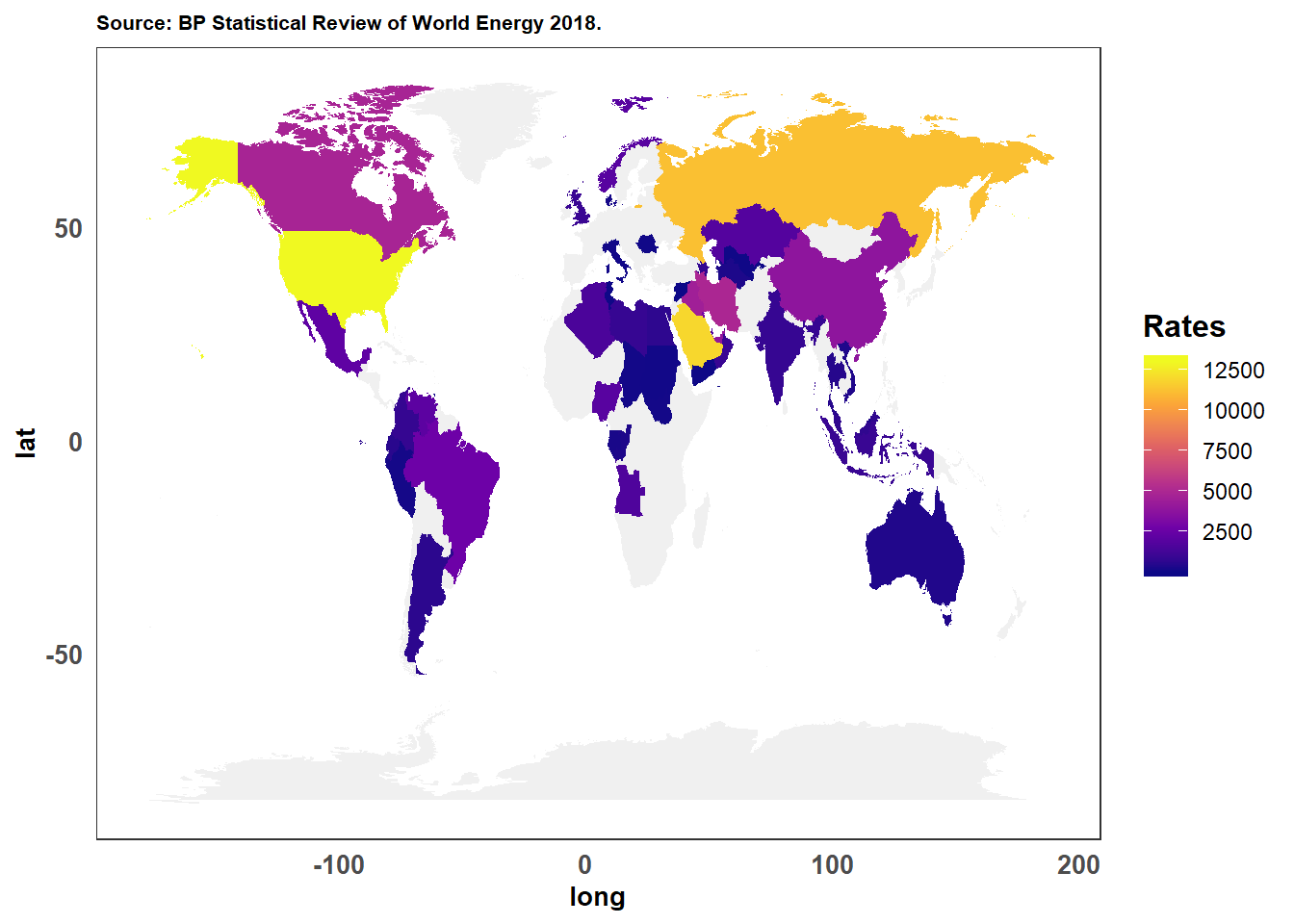World map of daily oil production rates in thousand of barrels per day in 2017. There is either no data or lumped data for gray-colored countries.

``````highest_production_2017 <- oil_production_long %>% filter(year == 2017) %>% select(country,oil_rate) %>% arrange(desc(oil_rate))

country_ordered <- factor(highest_production_2017\$country, ordered = TRUE, levels = highest_production_2017\$country)

highest_production_2017\$country <- country_ordered

ggplot(highest_production_2017[c(1:20),],aes(x = country,y = oil_rate, fill = country)) +
geom_col() +
scale_x_discrete(name = "") +
scale_y_continuous(name = "Oil production rate (Thousand barrels per day)", breaks = c(0, 1000,2000,3000,4000,5000,6000,7000,8000 ,9000,10000,11000,12000,13000)) +
ggtitle("Source: BP Statistical Review of World Energy 2018.") +
theme_bw() +
theme(axis.text = element_text(size = 10, face = "bold"),
axis.title = element_text(size = 10, face = "bold"),
axis.text.x = element_text(angle = 90, hjust = 1),
axis.ticks.x = element_blank(),
panel.grid  = element_blank(),
plot.title = element_text(size = 8, face = "bold"),
legend.position = "none") ``````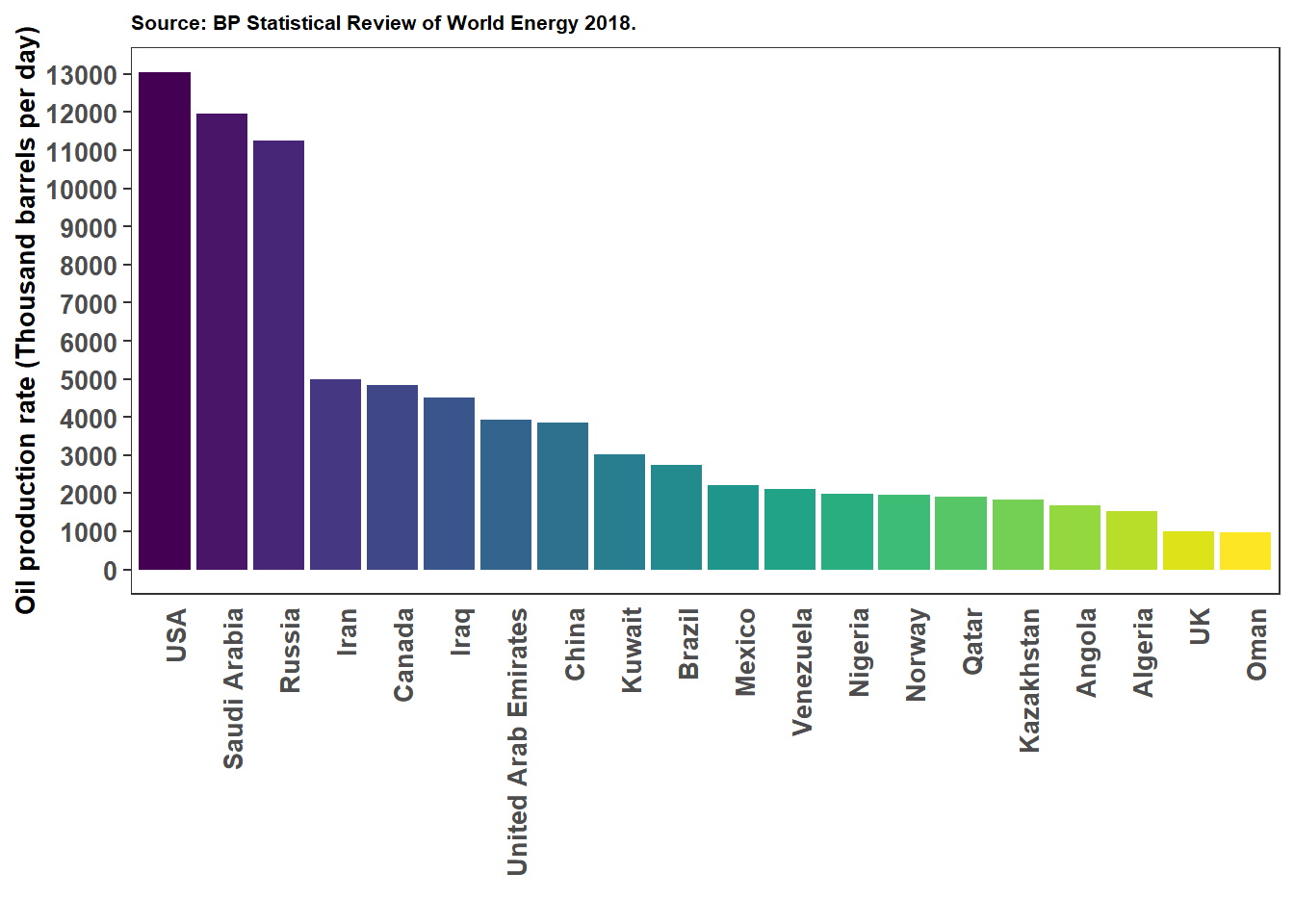The top 20 countries with the highest oil production rates at the end of 2017.

``````oil_production_long_region <- oil_production_long %>% group_by(Region_Continent, year) %>% summarise(total_prod = sum(oil_rate, na.rm = TRUE))

colbrew2 <- c("#e41a1c", "#377eb8", "#4daf4a", "#984ea3", "#ff7f00", "#ffff33", "#a65628")

ggplot(oil_production_long_region, aes(x = year, y = total_prod/1000, fill = Region_Continent)) +
geom_area() +
scale_color_manual(values = colbrew2) +
scale_x_continuous(name = "year", expand = c(0, 0), limits = c(1980, 2018), breaks = c(1980,1985,1990,1995,2000,2005,2010,2015,2018)) +
scale_y_continuous(name = "Oil production rate (Million barrels per day)", limits = c(0, 100), expand = c(0, 0), breaks = c(10,20,30,40,50,60,70,80,90,100)) +
ggtitle("Source: BP Statistical Review of World Energy 2018.
*CIS: Commonwealth of Independent States.") +
theme_bw() +
theme(axis.text = element_text(size = 10, face = "bold"),
axis.title = element_text(size = 10, face = "bold"),
legend.title = element_text(size = 12, face = "bold"),
panel.grid  = element_blank(),
plot.title = element_text(size = 8, face = "bold")) ``````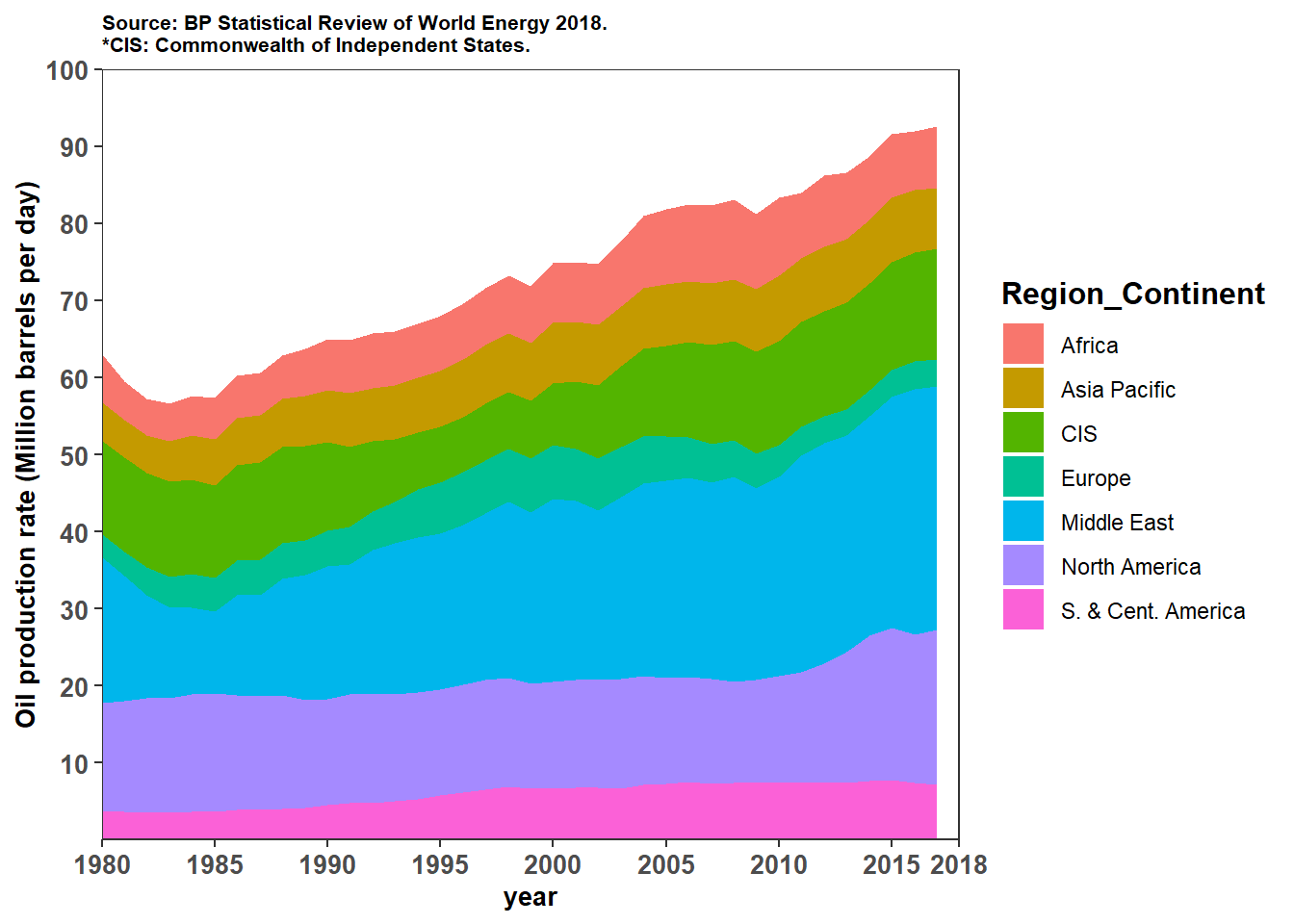Oil production rate vs time in different regions/continents.

#### 3.3. Oil consumption plots

``````oil_consumption <- as.data.frame(read_excel("bp_stats_review_2018_all_data.xlsx", sheet = "Oil Consumption - Barrels", range = "A3:BB109"))

names(oil_consumption) <- "country"

oil_consumption <- select(oil_consumption,1:(ncol(oil_consumption)))

oil_consumption <- oil_consumption[complete.cases(oil_consumption),]

Region_index <- which(str_detect(oil_consumption[,1] ,pattern = "Total"))

Region_name <- as.character(str_split(oil_consumption[Region_index,1],pattern = " ", n = 2, simplify = TRUE)[,2])

Region_info <- bind_cols(as.data.frame(Region_index),as.data.frame(Region_name,stringsAsFactors = FALSE))

counter <- 1

temp <- Region_index

for (i in (1:tail(Region_index,1))) {
if (i < temp) {
oil_consumption\$Region_Continent[i] <- as.character(Region_info[Region_index == temp,])
} else if (i == temp) {
counter <- counter  + 1
temp <- Region_index[counter]
oil_consumption\$Region_Continent[i] <- ""
} else {
}
}

# Wide format
oil_consumption <- oil_consumption[-Region_index,]

oil_consumption <- select(oil_consumption,country,Region_Continent,as.character(1980:2017))

oil_consumption[oil_consumption == "n/a"] <- NA

oil_consumption[3:ncol(oil_consumption)] <- as.numeric(unlist(oil_consumption[3:ncol(oil_consumption)]))

# Long format
oil_consumption_long <- oil_consumption %>% pivot_longer(names_to = "year", values_to = "oil_rate", -c("country","Region_Continent"))

oil_consumption_long\$year <- as.integer(oil_consumption_long\$year)``````
``````world_map <- ggplot2::map_data("world")

unique(oil_consumption_long\$country[!(oil_consumption_long\$country %in% world_map\$region)])``````
``````##   "US"                    "Trinidad & Tobago"
##   "Central America"       "Other Caribbean"
##   "Other South America"   "United Kingdom"
##   "Other Europe"          "Russian Federation"
##   "USSR"                  "Other CIS"
##  "Other Middle East"     "Eastern Africa"
##  "Middle Africa"         "Western Africa"
##  "Other Northern Africa" "Other Southern Africa"
##  "China Hong Kong SAR"   "Other Asia Pacific"``````
``````oil_consumption_long[oil_consumption_long\$country == "US",]\$country <- "USA"

oil_consumption_long[oil_consumption_long\$country == "United Kingdom",]\$country <- "UK"

oil_consumption_long[oil_consumption_long\$country == "Russian Federation",]\$country <- "Russia"

world_consumption <- left_join(world_map, oil_consumption_long, by = c('region' = 'country'))

world_consumption_2017 <- world_consumption %>% filter(year %in% c(NA,2017))

colnames(world_consumption_2017) <- "Rates"``````
``````ggplot() +
geom_map(data = world_consumption_2017, map = world_consumption_2017, aes(x = long, y = lat, map_id=region, fill = Rates)) +
scale_fill_continuous(low = "lightgreen", high = "darkgreen", guide = "colorbar",na.value = "gray93", limits = c(0,20000), breaks = c(0,5000,10000,15000,20000)) +
ggtitle("Source: BP Statistical Review of World Energy 2018.") +
theme_bw() +
theme(axis.text = element_text(size = 10, face = "bold"),
axis.title = element_text(size = 10, face = "bold"),
axis.ticks = element_blank(),
panel.grid  = element_blank(),
legend.title = element_text(size = 12, face = "bold"),
plot.title = element_text(size = 8, face = "bold"))``````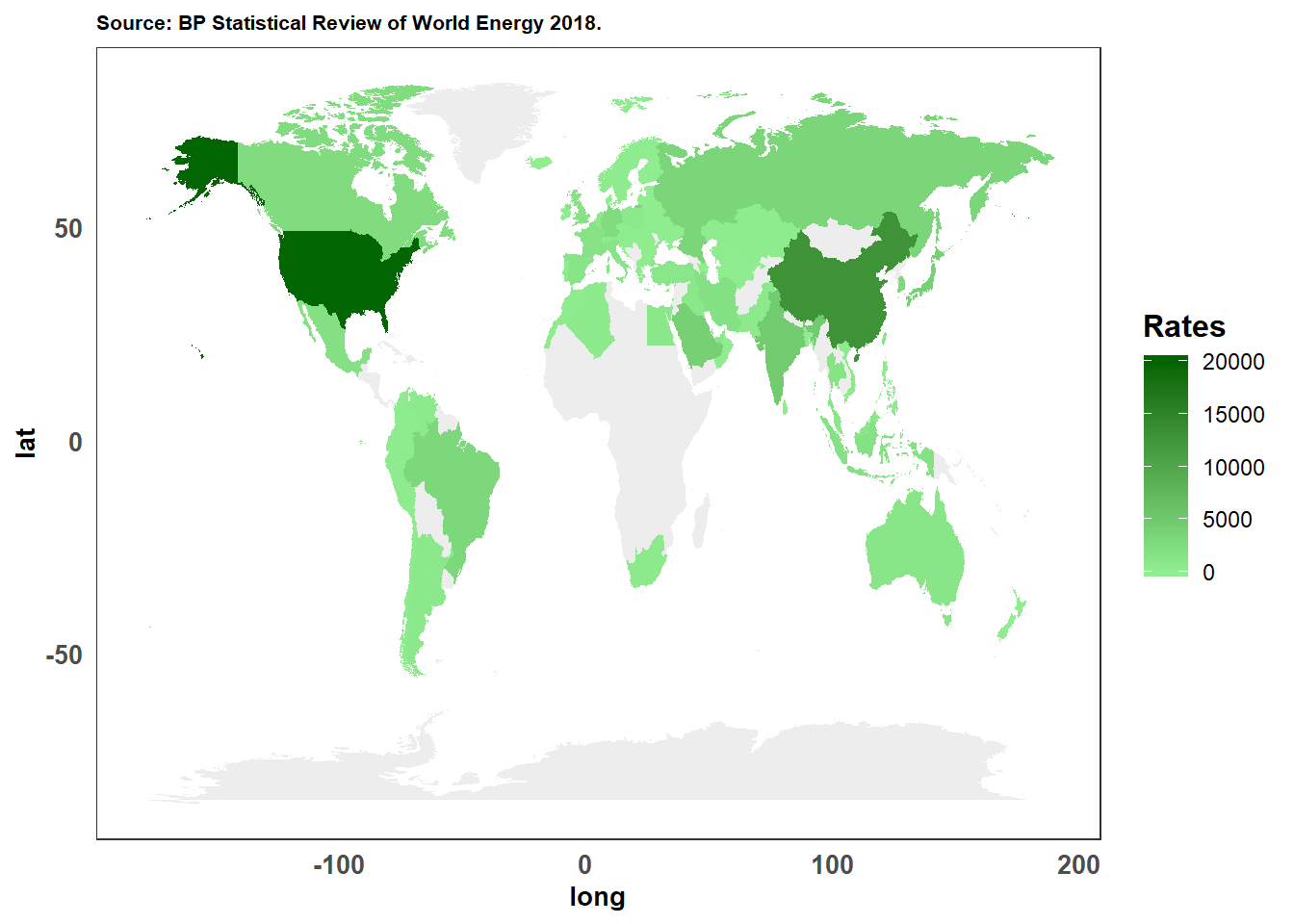World map of daily oil consumption rates in thousand of barrels per day in 2017. There is either no data or lumped data for gray-colored countries.

``````highest_consumption_2017 <- oil_consumption_long %>% filter(year == 2017) %>% select(country,oil_rate) %>% arrange(desc(oil_rate))

country_ordered <- factor(highest_consumption_2017\$country, ordered = TRUE, levels = highest_consumption_2017\$country)

highest_consumption_2017\$country <- country_ordered

ggplot(highest_consumption_2017[c(1:20),],aes(x = country,y = oil_rate, fill = country)) +
geom_col() +
scale_x_discrete(name = "") +
scale_y_continuous(name = "Oil consumption rate (Thousand barrels per day)", breaks = c(0, 1000,2000,3000,4000,5000,6000,7000,8000 ,9000,10000,11000,12000,13000,14000,15000,16000,17000,18000,19000,20000)) +
ggtitle("Source: BP Statistical Review of World Energy 2018.") +
theme_bw() +
theme(axis.text = element_text(size = 10, face = "bold"),
axis.title = element_text(size = 10, face = "bold"),
axis.text.x = element_text(angle = 90, hjust = 1),
axis.ticks.x = element_blank(),
panel.grid  = element_blank(),
plot.title = element_text(size = 8, face = "bold"),
legend.position = "none") ``````